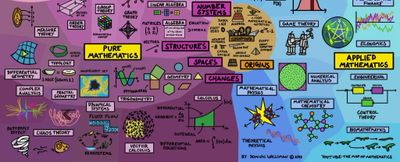Broadly speaking, pure mathematics  is mathematics that studies entirely abstract concepts

One central concept in pure mathematics is the idea of generality; pure mathematics often exhibits a trend towards increased generality. Uses and advantages of generality include the following:

• Generalizing theorems or mathematical structures can lead to deeper understanding of the original theorems or structures
• Generality can simplify the presentation of material, resulting in shorter proofs or arguments that are easier to follow.
• One can use generality to avoid duplication of effort, proving a general result instead of having to prove separate cases independently, or using results from other areas of mathematics.
• Generality can facilitate connections between different branches of mathematics. Category theory is one area of mathematics dedicated to exploring this commonality of structure as it plays out in some areas of math.

Pure mathematics is comprised of 4 major sub-branches:

1. Quantitative Mathematics starts with numbers, first the familiar natural numbers and integers ("whole numbers") and arithmetical operations on them, which are characterized in arithmetic.
2. Structural Mathematics (Abstract Algebra) deals with many mathematical objects, such as sets of numbers and functions which exhibit internal structure as a consequence of operations or relations that are defined on the set.
3. Spatial Mathematics starts with the study of space originating with geometry – in particular, Euclidean geometry, which combines space and numbers,
4. Mathematical Analysis (Change Mathematics) is the branch of mathematics dealing with limits and related theories, such as differentiation, integration, measure, infinite series, and analytic functions.#### 1. Quantitative Mathematics

Quantitative Mathematics starts with the quantity of numbers, first the familiar natural numbers and integers ("whole numbers") and arithmetical operations on them, which are characterized in arithmetic. The deeper properties of integers are studied in number theory, from which come such popular results as Fermat's Last Theorem. The twin prime conjecture and Goldbach's conjecture are two unsolved problems in number theory. As the number system is further developed, the integers are recognized as a subset of the rational numbers ("fractions"). These, in turn, are contained within the real numbers, which are used to represent continuous quantities. Real numbers are generalized to complex numbers. These are the first steps of a hierarchy of numbers that goes on to include quaternions and octonions. Consideration of the natural numbers also leads to the transfinite numbers, which formalize the concept of "infinity". According to the fundamental theorem of algebra all solutions of equations in one unknown with complex coefficients are complex numbers, regardless of degree. Another area of study is the size of sets, which is described with the cardinal numbers. These include the aleph numbers, which allow meaningful comparison of the size of infinitely large sets.

#### 2. Structural Mathematics ( Abstract Algebra )

In structural mathematics (Abstract Algebra), many mathematical objects, such as sets of numbers and functions, exhibit internal structure as a consequence of operations or relations that are defined on the set. Mathematics then studies properties of those sets that can be expressed in terms of that structure; for instance number theory studies properties of the set of integers that can be expressed in terms of arithmetic operations. Moreover, it frequently happens that different such structured sets (or structures) exhibit similar properties, which makes it possible, by a further step of abstraction, to state axioms for a class of structures, and then study at once the whole class of structures satisfying these axioms. Thus one can study groups, rings, fields and other abstract systems; together such studies (for structures defined by algebraic operations) constitute the domain of abstract algebra. By its great generality, abstract algebra can often be applied to seemingly unrelated problems; for instance a number of ancient problems concerning compass and straightedge constructions were finally solved using Galois theory, which involves field theory and group theory. Another example of an algebraic theory is linear algebra, which is the general study of vector spaces, whose elements called vectors have both quantity and direction, and can be used to model (relations between) points in space. This is one example of the phenomenon that the originally unrelated areas of geometry and algebra have very strong interactions in modern mathematics. Combinatorics studies ways of enumerating the number of objects that fit a given structure. Some areas of mathematics that fall under the classification abstract algebra have the word algebra in their name; linear algebra is one example. Others do not: group theory, ring theory, and field theory are examples.

#### 3. Spatial Mathematics

In spatial Mathematics the study of space originates with geometry – in particular, Euclidean geometry, which combines space and numbers, and encompasses the well-known Pythagorean theorem

#### 4. Change Mathematics (Analysis)

In change mathematics, understanding and describing change is a common theme in the natural sciences, and calculus was developed as a powerful tool to investigate it. Functions arise here, as a central concept describing a changing quantity. Mathematical analysis is the branch of mathematics dealing with limits and related theories, such as differentiation, integration, measure, infinite series, and analytic functions. These theories are usually studied in the context of real and complex numbers and functions. Analysis evolved from calculus, which involves the elementary concepts and techniques of analysis. Analysis may be distinguished from geometry; however, it can be applied to any space of mathematical objects that has a definition of nearness (a topological space) or specific distances between objects (a metric space).